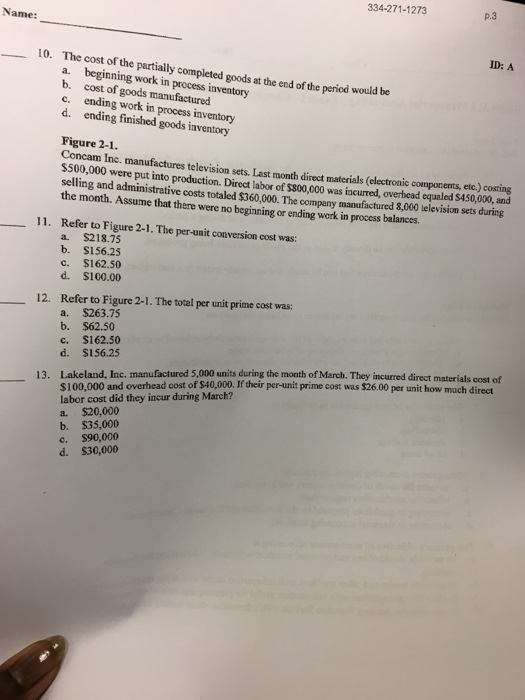# Question & Answer: The cost of the partially completed goods at the end of the period would be a. beginning work in process inventory b. cost of goods manufact…..The cost of the partially completed goods at the end of the period would be a. beginning work in process inventory b. cost of goods manufactured c. ending work in process inventory d. ending finished goods inventory Concam Inc. manufactures television sets. Last month direct materials (electronic components, etc.) costing \$500, 000 were put into production. Direct labor of \$800, 000 was incurred, overhead equaled \$450, 000, and selling and administrative costs totaled \$360, 000. The company manufactured 8, 000 television sets during the month. Assume that there were no beginning or ending work in process balances. Refer to Figure 2-1. The per-unit conversion cost was: a. \$218.75 b. \$156.25 c. \$162.50 d. S100.00 Refer to Figure 2-1. The total per unit prime cost was: a. \$263.75 b. \$62.50 c. \$162.50 d. \$156.25 Lakeland, Inc. manufactured 5, 000 units during the month of March. They incurred direct materials cost of \$100, 000 and overhead cost of \$40, 000. If their per-unit prime cost was \$26.00 per unit how much direct labor cost did they incur during March? a. \$20, 000 b. \$35, 000 c. \$90, 000 d 530, 000 13.

10) Cost of partially completed goods is a part of Closing work in process inventory as the goods are yet to be completed to be part of finished goods.
So, the answer is Option C

Don't use plagiarized sources. Get Your Custom Essay on
Question & Answer: The cost of the partially completed goods at the end of the period would be a. beginning work in process inventory b. cost of goods manufact…..
GET AN ESSAY WRITTEN FOR YOU FROM AS LOW AS \$13/PAGE

11) Conversion cost per unit = Direct labor + Manufacturing overheads/Number of units
\$800000+\$450000/8000 = \$1250000/8000 = \$156.25 per unit
Option B

12) Prime cost per unit = Direct material + Direct labor /Number of units
\$500000+\$800000/8000 units
= \$1300000/8000 units
= \$162.5
Option C

13) Total prime cost= 5000*\$26 = \$130000.
Prime cost = Direct material + Direct labor
Direct labor = Prime cost – Direct material
\$130000-\$100000
=\$30000
option D